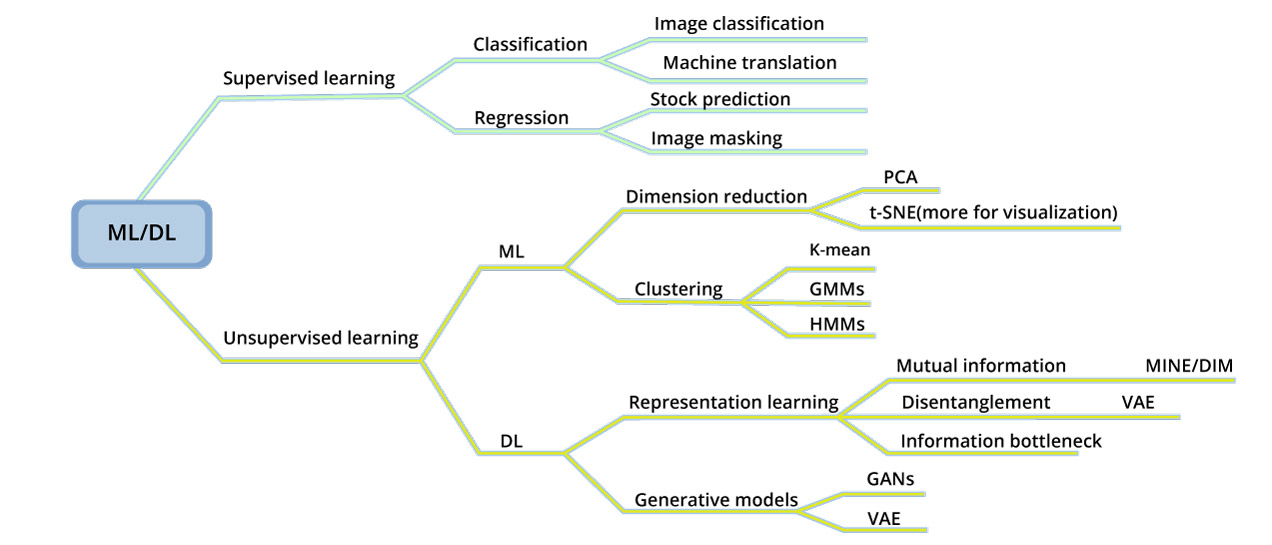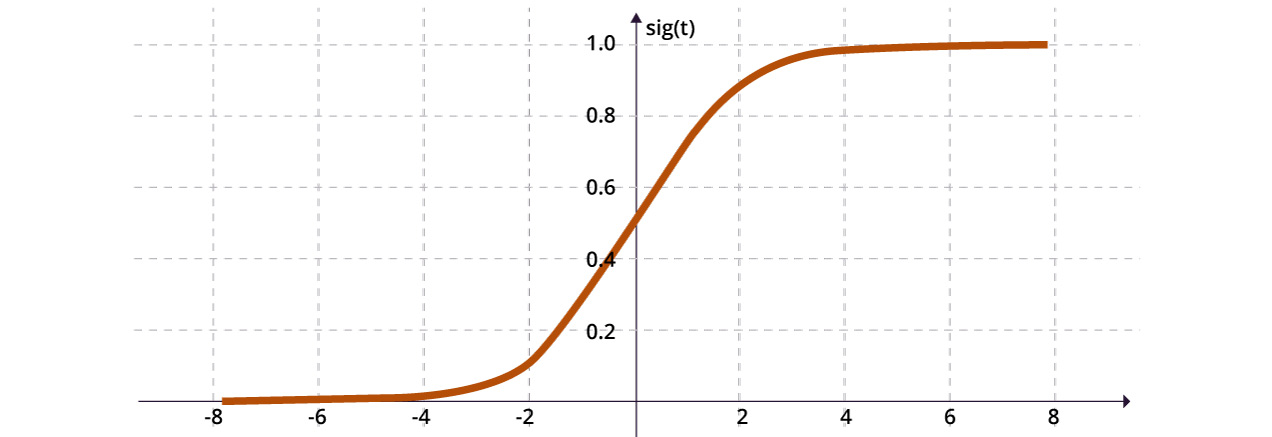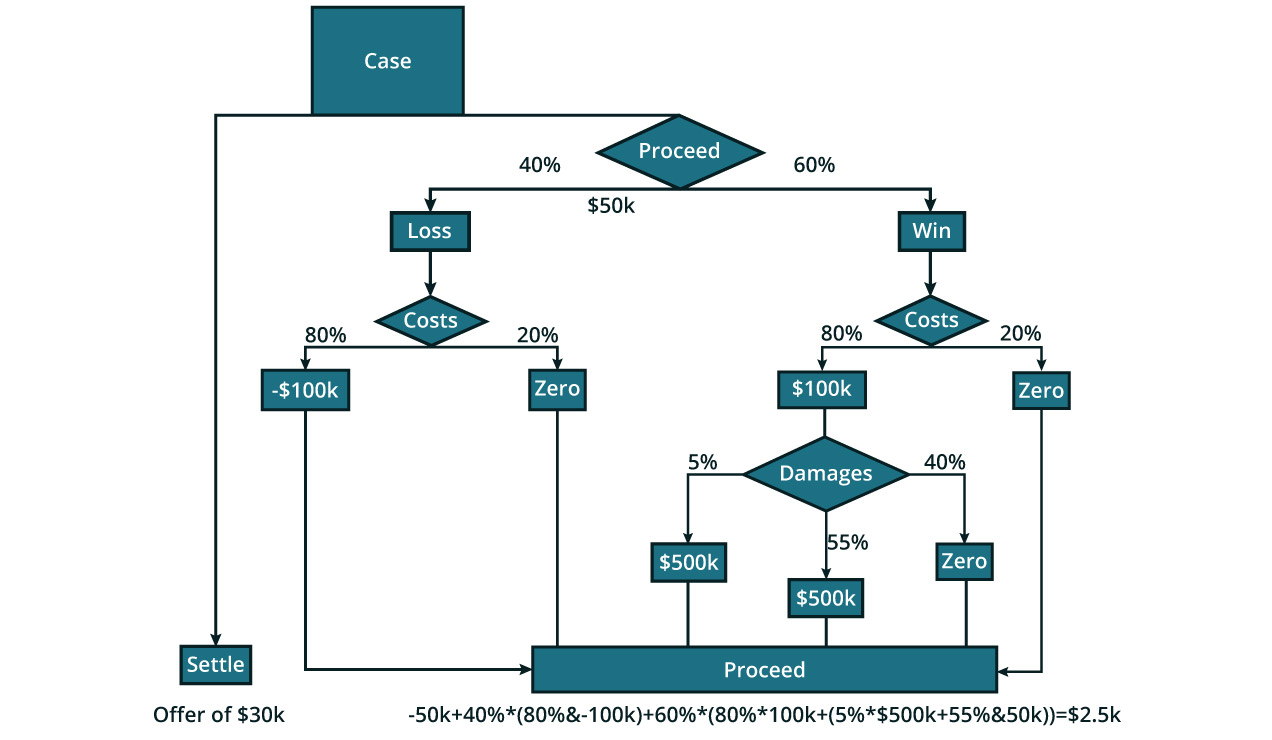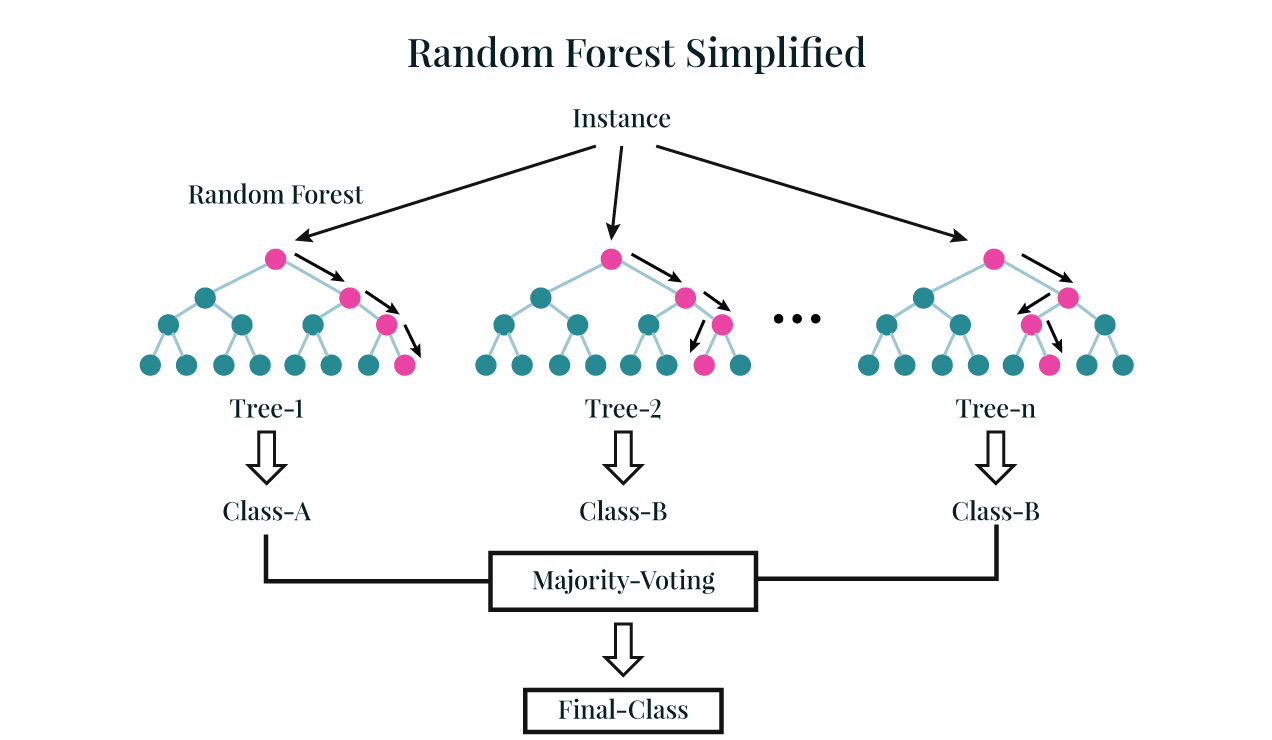# Essential Steps to Mastering Machine Learning with Python

One of the world’s most popular programming languages today, Python is a great tool for Machine Learning (ML) and Artificial Intelligence (AI). It is an open-source, reusable, general-purpose, object-oriented, and interpreted programming tool. Python’s key design ideology is code readability, ease of use and high productivity. The latest trend shows that the interest in Python has grown significantly over the past five years. Python is the top choice for ML/AI enthusiasts when compared to other programming languages.Image source: Google Trends – comparing Python with other tools in the market

## What makes Python a perfect recipe for Machine Learning?

Python can be used to write Machine Learning algorithms and it computes pretty accurately. Python’s concise and easy readability allows the writing of reliable code very quickly. Another reason for its popularity is the availability of various versatile, ready-to-use libraries.

It has an excellent library ecosystem and a great tool for developing prototypes. Unlike R, Python is a general-purpose programming language which can be used to build web applications and enterprise applications.

The community of Python has developed libraries that adhere to a particular area of data science application. For instance, there are libraries available for handling arrays, performing numerical computation with matrices, statistical computing, machine learning, data visualization and many more. These libraries are highly efficient and make the coding much easier with fewer lines of codes.

Let us have a brief look at some of the important Python libraries that are used for developing machine learning models.

• NumPy: One of the fundamental packages for numerical and scientific computing. It is a mathematical library to work with n-dimensional arrays in Python.
• Pandas: Provides highly efficient, easy-to-use DataFrame for DataFrame manipulations and Exploratory Data Analysis (EDA).
• SciPy: SciPy is a functional library for scientific and high-performance computations. It contains modules for optimization and for several statistical distributions and tests.
• Matplotlib: It is a complete plotting package that provides 2D plotting as well as 3D plotting. It can plot static and interactive plots.
• Seaborn: Seaborn library is based on Matplotlib. It is used to plot more elegant statistical visualization.
• StatsModels: The StatsModels library provides functionalities for estimation of various statistical models and conducting different statistical tests.
• Scikit-learn: Scikit-Learn is built on NumPy, SciPy and Matplotlib. Free to use, overpowered and provides various range of supervised and unsupervised machine learning algorithms.

One should also take into account the importance of IDEs specially designed for Python for Machine Learning.

The Jupyter Notebook  -  an open-source web-based application that enables ML enthusiasts to create, share, quote, visualize, and live-code their projects.

There are various other IDEs that can be used like PyCharm, Spyder, Vim, Visual Studio Code. For beginners, there is a nice simple online compiler available – Programiz.

## Roadmap to master Machine Learning Using Python

1. Learn Python: Learn Python from basic to advanced. Practice those features that are important for data analysis, statistical analysis and Machine Learning. Start from declaring variables, conditional statements, control flow statements, functions, collection objects, modules and packages. Deep dive into various libraries that are used for statistical analysis and building machine learning models.
2. Descriptive Analytics : Learn the concept of descriptive analytics, understand the data, learn to load structured data and perform Exploratory Data Analysis (EDA). Practice data filtering, ordering, grouping, multiple joining of datasets. Handle missing values, prepare visualization plots in 2D or 3D format (from libraries like seaborn, matplotlib) to find hidden information and insights.
3. Take a break from Python and Learn Stats  Learn the concept of the random variable and its important role in the field of analytics. Learn to draw insights from the measures of dispersion (mean, median, mode, quartiles and other statistical measures like confidence interval and distribution functions. The next step is to understand probability & various probability distributions and their crucial role in analytics. Understand the concept of various hypothesis tests like t-tests, z-test, ANOVA (Analysis of Variance), ANCOVA (Analysis of Covariance), MANOVA (Multivariate Analysis of Variance), MANCOVA (Multivariate Analysis of Covariance) and chi-square test.
4.  Understand Major Machine Learning AlgorithmsImage source

Different algorithms have different tasks. It is advisable to understand the context and select the right algorithm for the right task.

Types of ML Problem Description Examples
Classification Pick one of N labels Predict if loan is going to be defaulted or not
Regression Predict numerical values Predict property price
Clustering Group similar examples Most relevant documents
Association rule learning Infer likely association patterns in data If you buy butter you are likely to buy bread (unsupervised
Structured Output Create complex output Natural language parse trees, images recognition bounding boxes
Ranking Identify position on a scale or status Search result ranking

Source

A. Regression (Prediction):  Regression algorithms are used for predicting numeric values. For example, predicting property price, vehicle mileage, stock prices and so on.Source

B. Linear Regression – predicting a response variable, which is numeric in nature, using one or more features or variables. Linear regression model is mathematically represented as:Source

Various regression algorithms include:

• Linear Regression
• Polynomial Regression
• Exponential Regression
• Decision Tree
• Random Forest
• Neural Network

As a note to new learners, it is suggested to understand the concepts of – Regression assumptions, Ordinary Least Square Method, Dummy Variables (n-1 dummy encoding, one hot encoding), and performance evaluation metrics (RMSE, MSE, MAD).

• Classification – We use classification algorithms for predicting a set of items’ classes or a categorical feature. For example, predicting loan default (yes/no) or predicting cancer (yes/no) and so on.

Various classification algorithms include:

• Binomial Logistic Regression
• Fractional Binomial Regression
• Quasibinomial Logistic regression
• Decision Tree
• Random Forest
• Neural Networks
• K-Nearest Neighbor
• Support Vector Machines

Some of the classification algorithms are explained here:

• K-Nearest Neighbors – simple yet often used classification algorithm.
• It is a non-parametric algorithm (does not make any assumption on the underlying data distribution)
• It chooses to memorize the learning instances
• The output is a class membership
• There are three key elements in this approach – a set of labelled objects, eg, a set of stored records, a distance between objects, and the value of k, the number of nearest neighbours
• Distance measures that the K-NN algorithm uses – Euclidean distance (square root of the sum of the squared distance between a new point and the existing point across all the input attributes.

Other distances include – Hamming distance, Manhattan distance, Minkowski distanceSource

Example of K-NN classification. The test sample (green dot) should be classified either to blue squares or to red triangles. If k = 3 (solid line circle) it is assigned to the red triangles because there are 2 triangles and only 1 square inside the inner circle. In other words the number of triangles is more than the number of squares If k = 5 (dashed line circle) it is assigned to the blue squares (3 squares vs. 2 triangles inside the outer circle). It is to be noted that to avoid equal voting, the value of should be odd and not even.

• Logistic Regression – A supervised algorithm that is used for binary classification. The basis for logistic regression is the logit feature aka sigmoid characteristic which takes any real value and maps it between zero and 1. In other words, Logistic Regression returns a probability value for the class label.Source

1. If the output of the sigmoid function is more than 0.5, we can classify the outcome as 1 or YES, and if it is less than 0.5, we can classify it as 0 or NO

1. For instance, let us take cancer prediction. If the output of the Logistic Regression is 0.75, we can say in terms of probability that, “There is a 75 percent chance that the patient will suffer from cancer.”

Decision Tree – Is a type of supervised learning algorithm which is most commonly used in the case of a classification problem. Decision Tree algorithms can also be used for regression problems i.e. to predict a numerical response variable. In other words, Decision Tree works for both categorical and continuous input and output variables.

• Each branch node of the decision tree represents a choice between some alternatives and each leaf node represents a decision.Source

As an early learner, it is suggested to understand the concept of ID3 algorithm, Gini Index, Entropy, Information Gain, Standard Deviation and Standard Deviation Reduction.

• Random Forest – is a collection of multiple decision trees. It is a supervised learning algorithm, that can be used for both classification & regression problems. While algorithms like Decision Tree can cause a problem of overfitting wherein a model performs well in training data but does not perform well in testing or unseen data, algorithms like Random Forest can help avoid overfitting.

• It achieves uncorrelated decision trees throughout the concept of bootstrapping (i.e. sampling with replacement) and features randomness.Source

As a new learner it is important to understand the concept of bootstrapping.

• Support Vector Machine – a supervised learning algorithm, used for classification problems. Another flavour of Support Vector Machines (SVM) is Support Vector Regressor (SVR) which can be used for regression problems.

• In this, we plot each data item as a point in n-dimensional space
• n here represents the number of featuresSource

The value of each feature is the value of a particular coordinate.

Classification is performed by finding hyperplanes that differentiate the two classes.

It is important to understand the concept of margin, support vectors, hyperplanes and tuning hyper-parameters (kernel, regularization, gamma, margin). Also get to know various types of kernels like linear kernel, radial basis function kernel and polynomial kernel

• Naive Bayes – a supervised learning classifier which assumes features are independent and there is no correlation between them. The idea behind Naïve Bayes algorithm is the Bayes theorem.Source

### C.Clustering

Clustering algorithms are unsupervised algorithms that are used for dividing data points into groups such that the data points in each group are similar to each other and very different from other groups.

Some of the clustering algorithms include:

• K-means – An unsupervised learning algorithm in which the items are grouped into k-cluster

• The elements of the cluster are similar or homogenous.
• Euclidean distance is used to calculate the distance between two data points.
• Data points have a centroid; this centroid represents the cluster.
• The objective is to minimize the intra-cluster variations or the squared error function.SourceOther types of clustering algorithms:

• DBSCAN
• Mean Shift
• Hierarchical

### d) Association

Association algorithms, which form part of unsupervised learning algorithms, are for associating co-occurring items or events. Association algorithms are rule-based methods for finding out interesting relationships in large sets of data. For example, find out a relationship between products that are being bought together – say, people who buy butter also buy bread.

Some of the association algorithms are:

• Apriori Rules – Most popular algorithm for mining strong associations between variables. To understand how this algorithm works, concepts like Support, Confidence & Lift to be studied.
• ECLAT – Equivalence Class Clustering and bottom-up Lattice Traversal. This is one of the popular algorithms that is used for association problems. This algorithm is an enhanced version of the Apriori algorithm and is more efficient.
• FP Growth – Frequent Pattern Growth Algorithm – Another very efficient & scalable algorithm for mining associations between variables

### e) Anomaly Detection

We recommend the use of anomaly detection for discovering abnormal activities and unusual cases like fraud detection.

An algorithm that can be used for anomaly detection:

• Isolation Forest - This is an unsupervised algorithm that can help isolate anomalies from huge volume of data thereby enabling anomaly detection

### f) Sequence Pattern Mining

We use sequential pattern mining for predicting the next data events between data examples in a sequence.

• Predicting the next dose of medicine for a patient

### g) Dimensionality Reduction

Dimensionality reduction is used for reducing the dimension of the original data. The idea is to reduce the set of random features by obtaining a set of principal components or features. The key thing to understand in this is that the components retain or represent some meaningful properties of the original data. It can be divided into feature extraction and selection.

Algorithms that can be used for dimensionality reduction are:Source

Principal Component Analysis – This is a dimensionality reduction algorithm that is used to reduce the number of dimensions or variables in large datasets that have a very high number of variables. However it is to be noted that though PCA transforms a very large set of features or variables into smaller sets, it helps retain most of the information of the dataset. While the reduction of dimensions comes at a cost of model accuracy, the idea is to bring in simplicity in the model by reducing the number of variables or dimensions.

### h) Recommendation Systems –

Recommender Systems are used to build recommendation engines. Recommender algorithms are used in various business areas that include online stores to recommend the right product to its buyers like Amazon , content recommendation for online video & music sites like Netflix, Amazon Prime Music and various social media platforms like FaceBook, Twitter and so on.Source

Recommender Engines can be broadly categorized into the following types:

• Content-based methods — recommends items to a user based on their profile history. It revolves around customer’s taste and preference.
• Collaborating filtering method — it can be further subdivided into two categories

• Model-based — a stipulation wherein user and item interact. Both user and item interaction are learned from interactions matrix.
• Memory-based — Unlike model-based it relies on the similarity between the users and the items.
• Hybrid methods — Mix content which is based on collaborative filtering approaches.

Examples:

1. Movie recommendation system
2. Food recommendation system
3. E-commerce recommendation system

5. Choose the Algorithm — Several machine learning models can be used with the given context. These models are chosen depending on the data (image, numerical values, texts, sounds) and the data distribution

6. Train the model — Training the model is a process in which the machine learns from the historical data and provides a mathematical model that can be used for prediction. Different algorithms use different computation methods to compute the weights for each of the variables. Some algorithms like Neural Network initialize the weight of the variables at random. These weights are the values which affect the relationship between the actual and the predicted values.

7. Evaluation metrics to evaluate the model— Evaluation process comprises understanding the output model and evaluating the model accuracy for the result. There are various metrics to evaluate model performance. Regression problems have various metrics like MSE, RMSE, MAD, MAPE as key evaluation metrics while classification problems have metrics like Confusion Matrix, Accuracy, Sensitivity (True Positive Rate), Specificity (True Negative Rate), AUC (Area under ROC Curve), Kappa Value and so on.

It is only after the evaluation, the model can be improved or fine-tuned to get more accurate predictions. It is important to know a few more concepts like:

• True Positive
• True Negative
• False Positive
• False Negative
• Confusion Matrix
• Recall (R)
• F1 Score
• ROC
• AUC
• Log loss

When we talk about regression the most commonly used regression metrics are:

• Mean Absolute Error (MAE)
• Mean Squared Error (MSE)
• Root Mean Squared Error (RMSE)
• Root Mean Squared Logarithmic Error (RMSLE)
• Mean Percentage Error (MPE)
• Mean Absolute Percentage Error (MAPE)

We must know when to use which metric. It depends on the kind of data and the target variable you have.

8. Tweaking the model or the hyperparameter tuning  – With great models, comes the great problem of optimizing hyperparameters to build an improved and accurate ML model. Tuning certain parameters (which are called hyperparameters) is important to ensure improved performance. The hyperparameters vary from algorithm to algorithm and it is important to learn the hyperparameters for each algorithm.

9. Making predictions  – The final nail to the coffin. With all these aforementioned steps followed one can tackle real-life problems with advanced Machine Learning models.

Steps to remember while building the ML model:

• Data assembling or data collection  – generally represents the data in the form of the dataset.
• Data preparation – understanding the problem statement. This includes data wrangling for building or training models, data cleaning, removing duplicates, checking for missing values, data visualization for understanding the relationship between variables, checking for (imbalanced) bias data, and other exploratory data analysis. It also includes splitting the data into train and test.
• Choosing the model  –  the ML model which answers the problem statement. Different algorithms serve different purposes.
• Training the model  –  the idea to train the model is to ensure that the prediction is accurate more often.
• Model evaluation — evaluation metric to measure the performance of the model. How does the model perform against the previously unseen data? The train/test splitting ratio — (70:30) or (80:20), depending on the dataset. There is no exact rule to split the data by (80:20) or (70:30); it depends on the data and the target variable. Some of the data scientists use a range of 60% to 80% for training and the rest for testing the model.
• Parameter tuning  – to ensure improved performance by controlling the model’s learning process. The hyperparameters have to be tuned so that the model can optimally solve the machine learning problem. For parameter tuning, we either specify a grid of parameters known as the grid search or we randomly select a combination of parameters known as the random search.

• GridSearchCV — It is the process to search the best combination of parameters over the grid. For instance, n_estimator could possibly be 100,250,350,500; max_depth can be 2,5,11,15 and the criterion could be gini or entropy. Though these don’t look like a lot of parameters, just imagine the scenario if the dataset is too large. The grid search has to run on a loop and calculate the score on the validation set.
• RandomSearchCV —We randomly select a combination of parameters and then calculate the cross-validation score. It computes faster than GridSearch.

Note: Cross-validation is the first and most essential step when it comes to building ML models. If the cross-validation score is good, we can say that the validation data is a representation of training or the real-world data.

• Finally, making predictions — using the test data, of how the model will perform in real-world cases.

## Conclusion

Python has an extensive catalogue of modules and frameworks. It is fast, less complex and thus it saves development time and cost. It makes the program completely readable particularly for novice users. This particular feature makes Python an ideal recipe for Machine Learning.

Both Machine Learning and Deep Learning require work on complex algorithms and several workflows. When using Python, the developer can worry less about the coding, and can focus more on finding the solution. It is open-source and has an abundance of available resources and step-by-step documentation. It also has an active community of developers who are open to knowledge sharing and networking. The benefits and the ease of coding makes Python the go to choice for developers. We saw how Python has an edge over other programming tools, and why knowledge of Python is essential for ML right now.

Summing up we saw the benefits of Python, the way ahead for beginners and finally the steps required in a machine learning project. This article can be considered as a roadmap to your mastery over Machine Learning.